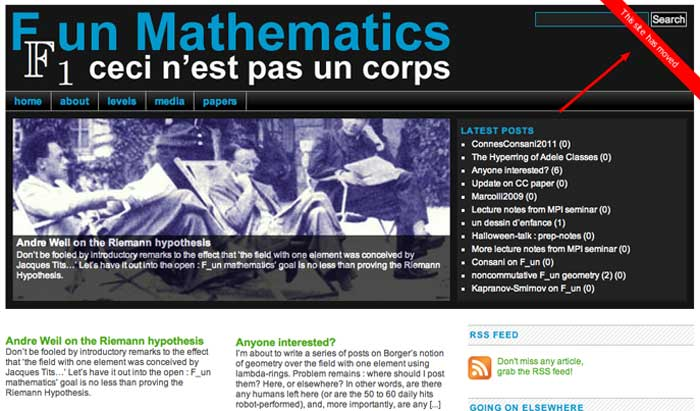# neverendingbooks Posts

These three ideas (re)surfaced over the last two decades, claiming to have potential applications to major open problems:

• (2000) $\mathbb{F}_1$-geometry tries to view $\mathbf{Spec}(\mathbb{Z})$ as a curve over the field with one element, and mimic Weil’s proof of RH for curves over finite fields to prove the Riemann hypothesis.
• (2012) IUTT, for Inter Universal Teichmuller Theory, the machinery behind Mochizuki’s claimed proof of the ABC-conjecture.
• (2014) topos theory : Connes and Consani redirected their RH-attack using arithmetic sites, while Lafforgue advocated the use of Caramello’s bridges for unification, in particular the Langlands programme.

It is difficult to voice an opinion about the (presumed) current state of such projects without being accused of being either a believer or a skeptic, resorting to group-think or being overly critical.

We lack the vocabulary to talk about the different phases a mathematical idea might be in.

Such a vocabulary exists in (information) technology, the five phases of the Gartner hype cycle to represent the maturity, adoption, and social application of a certain technology :

1. Technology Trigger
2. Peak of Inflated Expectations
3. Trough of Disillusionment
4. Slope of Enlightenment
5. Plateau of ProductivityThis model can then be used to gauge in which phase several emerging technologies are, and to estimate the time it will take them to reach the stable plateau of productivity. Here’s Gartner’s recent Hype Cycle for emerging Artificial Intelligence technologies.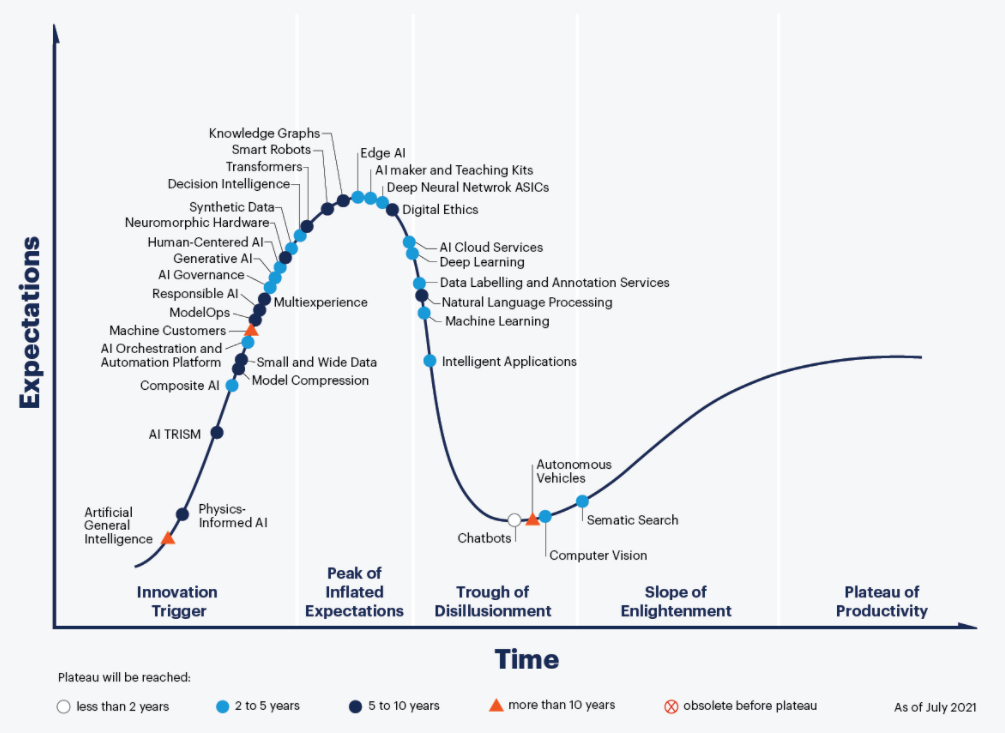Picture from Gartner Hype Cycle for AI 2021

What might these phases be in the hype cycle of a mathematical idea?

1. Technology Trigger: a new idea or analogy is dreamed up, marketed to be the new approach to that problem. A small group of enthusiasts embraces the idea, and tries to supply proper definitions and the very first results.
2. Peak of Inflated Expectations: the idea spreads via talks, blogposts, mathoverflow and twitter, and now has enough visibility to justify the first conferences devoted to it. However, all this activity does not result in major breakthroughs and doubt creeps in.
3. Trough of Disillusionment: the project ran out of steam. It becomes clear that existing theories will not lead to a solution of the motivating problem. Attempts by key people to keep the idea alive (by lengthy papers, regular meetings or seminars) no longer attract new people to the field.
4. Slope of Enlightenment: the optimistic scenario. One abandons the original aim, ditches the myriad of theories leading nowhere, regroups and focusses on the better ideas the project delivered.

A negative scenario is equally possible. Apart for a few die-hards the idea is abandoned, and on its way to the graveyard of forgotten ideas.

5. Plateau of Productivity: the polished surviving theory has applications in other branches and becomes a solid tool in mathematics.

It would be fun so see more knowledgable people draw such a hype cycle graph for recent trends in mathematics.

Here’s my own (feeble) attempt to gauge where the three ideas mentioned at the start are in their cycles, and here’s why:

• IUTT: recent work of Kirti Joshi, for example this, and this, and that, draws from IUTT while using conventional language and not making exaggerated claims.
• $\mathbb{F}_1$: the preliminary programme of their seminar shows little evidence the $\mathbb{F}_1$-community learned from the past 20 years.
• Topos: Developing more general theory is not the way ahead, but concrete examples may carry surprises, even though Gabriel’s topos will remain elusive.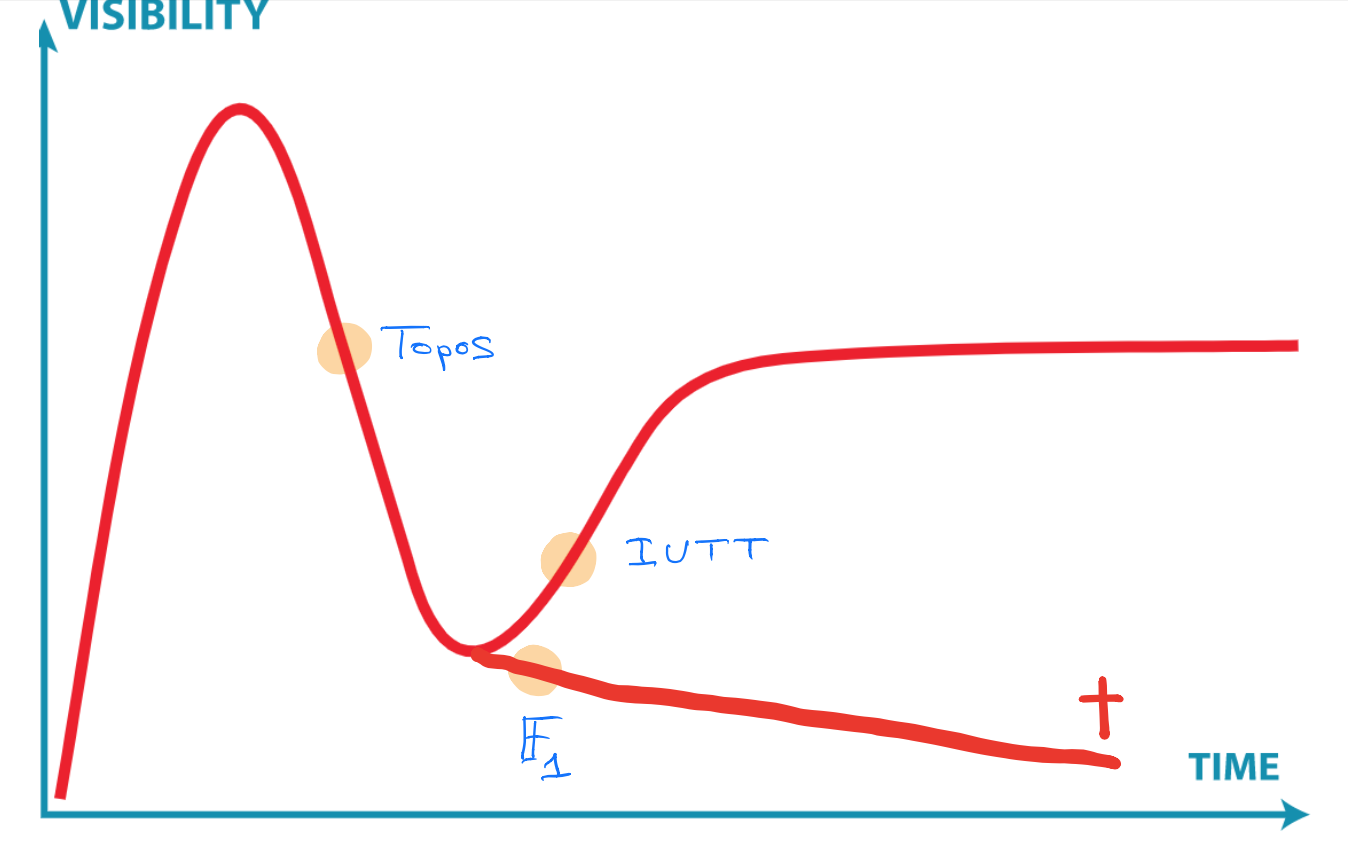Clearly, you don’t agree, and that’s fine. We now have a common terminology, and you can point me to results or events I must have missed, forcing me to redraw my graph.

Last week, Danielle Couty ArXiVed her paper Friendly views on Claude Chevalley (in French).

From the abstract: “We propose to follow the itinerary of Claude Chevalley during the last twenty years of his life, through the words of Jacques Roubaud, Denis Guedj and Alexander Grothendieck. Our perspective is that of their testimonies filled with friendship.”

Claude Chevalley was one of the founding fathers of Bourbaki. Two of the four pre-WW2 Bourbaki-congresses were held in “La Massoterie”, the Chevalley family domain in Chancay (see this post, update: later I learned from Liliane Beaulieu that the original house was destroyed by fire).

In 1938 he left for Princeton and stayed there during the war, making it impossible to return to a position in France for a very long time. Only in 1957 he could return to Paris where he led a seminar which proved to be essential for the development of algebraic groups and algebraic geometry.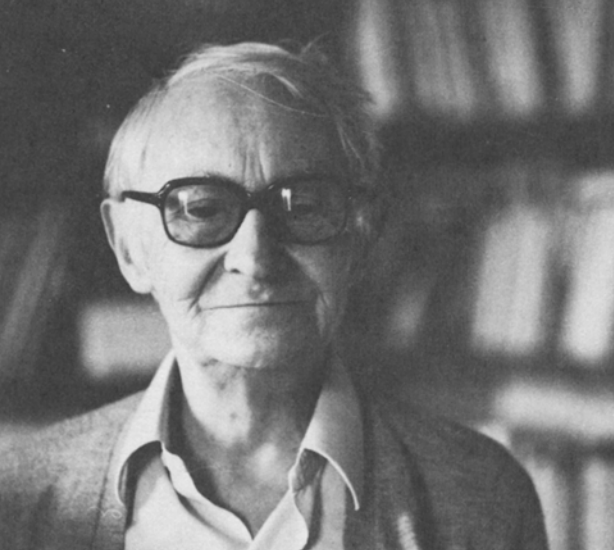Picture from N. Bourbaki, an interview with C. Chevalley

The Couty paper focusses on the post-1968 period in which Chevalley distanced himself from Bourbaki (some of its members, he thought, had become ‘mandarins’ and ‘reactionaires’), became involved with the ecological movement ‘Survivre et vivre’ and started up the maths department of a new university at Vincennes.

The paper is based on the recollections of three of his friends.

1. Jacques Roubaud is a French poet, writer and mathematician.

On this blog you may have run into Roubaud as the inventor of Bourbaki’s death announcement, and the writer of the book with title $\in$.

He’s also a member of Oulipo, a loose gathering of (mainly) French-speaking writers and mathematicians. Famous writers such as Georges Perec and Italo Calvino were also Oulipo-members (see also Ouilpo’s use of the Tohoku paper).

Chevalley introduced Roubaud (and others) to the game of Go. From Couty’s paper this quote from Roubaud (G-translated):

“. . . it turns out that he had learned to play go in Japan and then, in Paris, he could not find a player […] I played go with him […] and then at a certain moment , we thought, Pierre Lusson and myself, it would still be good to create circumstances such that Chevalley could have players. And so, we had a lot of ambition, we said to ourselves: “We’re going to write a treatise on go, and then lots of people will start playing go”. »

The resulting Go-book is A short treatise inviting the reader to discover the subtle art of Go. Here’s Georges Perec (left) and Jacques Roubaud playing a game.Picture from Petit traite invitant a la decouverte de l’art subtil du Go

2. Denis Guedj was a French novelist, mathematician and historian of science professor, perhaps best known for his book The Parrot’s Theorem.

In May 1968, Guedj was a PhD-student of Jean-Paul Benzecri (the one defining God as the Alexandroff compactification of the univers), working in the building where ‘Le Comité de Grève’ installed itself. Here he met Chevalley. A Guedj-quote from Couty’s paper (G-translated):

“Claude Chevalley was one of the three professors of the Faculty of Science to commit himself totally to the adventure until the end, occupying the premises with the students on the Quai Saint-Bernard […] and sleeping there frequently . That’s where I met him.

We had taken possession of this universe which until then had only been a place of study and knowledge, and which, in the mildness of this month of May, had become a place of life, of a life wonderfully exhilarating. The college was ours. At night we walked down the aisles yet? lined with tall trees, entered the empty lecture halls, slept under the stars. Needless to say that at the beginning of the school year, in the fall of 1968, it was impossible for us to find our place in these undressed spaces from which the magic had withdrawn. »Picture from Décès de l’écrivain et universitaire Denis Guedj

In June 2008, Guedj was one of the guests at the special edition of France Culture on the occasion of Grothendieck’s 80th birthday, Autour d’Alexandre Grothendieck.

3. Alexander Grothendieck, mathematician and misogynist, deified by some of today’s ‘mandarins’.

The paper by Danielle Couty may shed additional light on Grothendieck’s withdrawal from Bourbaki and mathematics as a whole. A G-translated Grothendieck quote from the paper:

“It was Chevalley who was one of the first, with Denis Guedj whom I also met through Survivre, to draw my attention to this ideology (they called it “meritocracy” or a name like that), and what there was in her of violence, of contempt. It was because of that, Chevalley told me […] that he could no longer bear the atmosphere in Bourbaki and had stopped setting foot there. »

Claude Chevalley stayed at Vincennes until his retirement in 1978, he died on June 28th 1984.

I met Christine in the late 80ties at some representation meeting in Oberwolfach. Christine was a regular at such meetings, being in the Michler-clique from Essen. I don’t recall why I was invited.

We had a fun time, and had a sneaky plan to be invited more regularly to the same conferences. All we had to do was to prove a good result, together…

Easier said than done. Christine’s field was modular representation theory (over $\overline{\mathbb{F}_p}$), and I was interested in the geometry of quiver moduli-spaces (over $\mathbb{C}$).

The next year I ran a post-graduate course on rationality problems and emailed the notes weekly to Christine. After all, results of Lenstra, Colliot-Thelene and Sansuc reduced the problem of (stable) rationality of algebraic tori to integral representation theory, a half-way meeting ground for both of us.

Around that time, our youngest daughter was born, and Christine graciously accepted to be her godmother.
Over the next years, she and Klaus visited us in Antwerp and we week-ended in their brand new house in the outskirts of Duisburg, close to a lake.Christine in Oberwolfach

Christine and I were working on the rationality problem for matrix invariants.

A sufficiently general $n \times n$ matrix is diagonalisable and is therefore determined, up to conjugacy, by $n$ free parameters (the eigenvalues), so the corresponding quotient-variety is rational.

Now consider couples of $n \times n$ matrices under simultaneous conjugation. In the mid 80ties, Formanek proved rationality for $n=3$ and $n=4$, by using the theory of algebraic tori, and that was about all that was known.

We were able to reduce the question of stable rationality for $n=5$ and $n=7$ to modular representation theory, after which Christine performed her magic to crack the problem. The paper appeared a year later in Inventiones.

Thirty years later, it is still the best result on rationality of matrix invariants.

So, we had our joint result, but its intended use never happened, and our contacts gradually watered down as our mathematical interests again diverged.

My thoughts go out to Klaus and all her loved ones.

January 13th, Gallimard published Grothendieck’s text Recoltes et Semailles in a fancy box containing two books.Here’s a G-translation of Gallimard’s blurb:

“Considered the mathematical genius of the second half of the 20th century, Alexandre Grothendieck is the author of Récoltes et semailles, a kind of “monster” of more than a thousand pages, according to his own words. The mythical typescript, which opens with a sharp criticism of the ethics of mathematicians, will take the reader into the intimate territories of a spiritual experience after having initiated him into radical ecology.

In this literary braid, several stories intertwine, “a journey to discover a past; a meditation on existence; a picture of the mores of a milieu and an era (or the picture of the insidious and implacable shift from one era to another…); an investigation (almost police at times, and at others bordering on the swashbuckling novel in the depths of the mathematical megapolis…); a vast mathematical digression (which will sow more than one…); […] a diary ; a psychology of discovery and creation; an indictment (ruthless, as it should be…), even a settling of accounts in “the beautiful mathematical world” (and without giving gifts…)”.”

All literary events, great or small, are cause for the French to fill a radio show.

January 21st, ‘Le grand entretien’ on France Inter invited Cedric Villani and Jean-Pierre Bourguignon to talk about Grothendieck’s influence on mathematics (h/t Isar Stubbe).

The embedded YouTube above starts at 12:06, when Bourguignon describes Grothendieck’s main achievements.

Clearly, he starts off with the notion of schemes which, he says, proved to be decisive in the further development of algebraic geometry. Five years ago, I guess he would have continued mentioning FLT and other striking results, impossible to prove without scheme theory.

Now, he goes on saying that Grothendieck laid the basis of topos theory (“to define it, I would need not one minute and a half but a year and a half”), which is only now showing its first applications.

Grothendieck, Bourguignon goes on, was the first to envision the true potential of this theory, which we should take very seriously according to people like Lafforgue and Connes, and which will have applications in fields far from algebraic geometry.

Topos20 is spreading rapidly among French mathematicians. We’ll have to await further results before Topos20 will become a pandemic.

Another interesting fragment starts at 16:19 and concerns Grothendieck’s gribouillis, the 50.000 pages of scribblings found in Lasserre after his death.

Bourguignon had the opportunity to see them some time ago, and when asked to describe them he tells they are in ‘caisses’ stacked in a ‘libraire’.

Here’s a picture of these crates taken by Leila Schneps in Lasserre around the time of Grothendieck’s funeral.If you want to know what’s in these notes, and how they ended up at that place in Paris, you might want to read this and that post.

If Bourguignon had to consult these notes at the Librairie Alain Brieux, it seems that there is no progress in the negotiations with Grothendieck’s children to make them public, or at least accessible.

Brendan Fong, David Spivak and Remy Tuyeras cooked up a vast generalisation of neural networks in their paper Backprop as Functor: A compositional perspective on supervised learning.

Here’s a nice introduction to neural networks for category theorists by Bruno Gavranovic. At 1.49m he tries to explain supervised learning with neural networks in one slide. Learners show up later in the talk.

$\mathbf{Poly}$ is the category of all polynomial functors, that is, things of the form
$p = \sum_{i \in p(1)} y^{p[i]}~:~\mathbf{Sets} \rightarrow \mathbf{Sets} \qquad S \mapsto \bigsqcup_{i \in p(1)} Maps(p[i],S)$
with $p(1)$ and all $p[i]$ sets.

Last time I gave Spivak’s ‘corolla’ picture to think about such functors.

I prefer to view $p \in \mathbf{Poly}$ as an horribly discrete ‘sheaf’ $\mathcal{P}$ over the ‘space’ $p(1)$ with stalk $p[i]=\mathcal{P}_i$ at point $i \in p(1)$.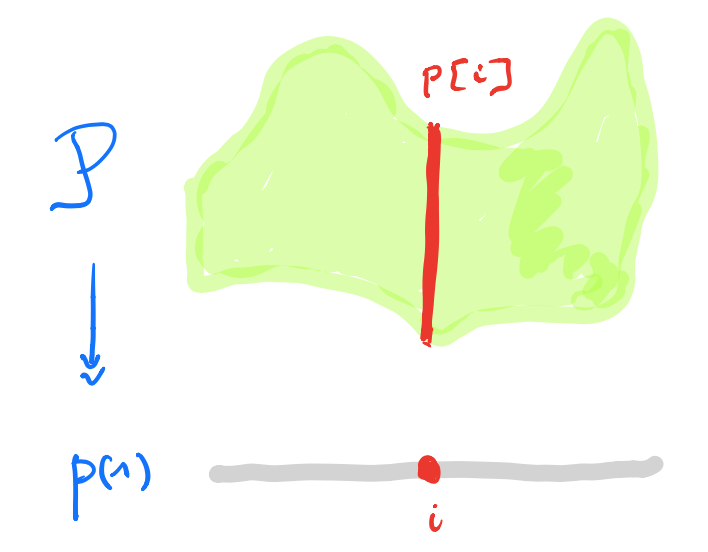A morphism $p \rightarrow q$ in $\mathbf{Poly}$ is a map $\varphi_1 : p(1) \rightarrow q(1)$, together with for all $i \in p(1)$ a map $\varphi^{\#}_i : q[\varphi_1(i)] \rightarrow p[i]$.

In the sheaf picture, this gives a map of sheaves over the space $p(1)$ from the inverse image sheaf $\varphi_1^* \mathcal{Q}$ to $\mathcal{P}$.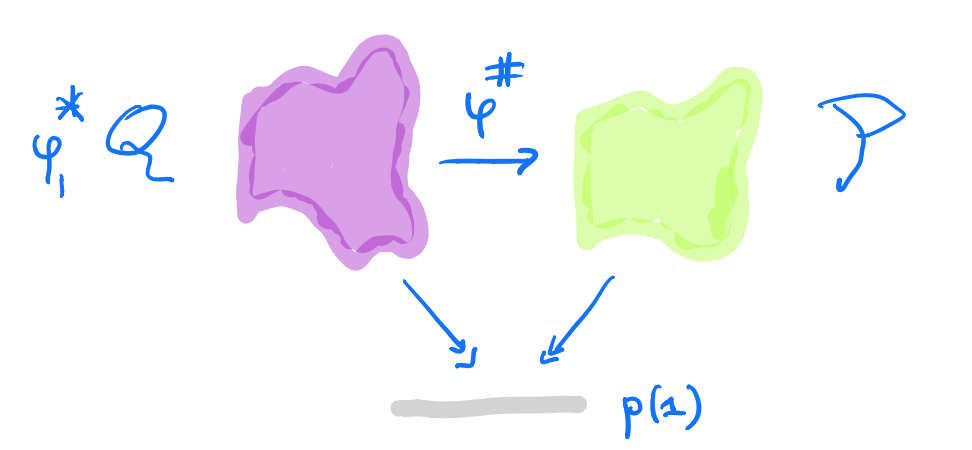But, unless you dream of sheaves in the night, by all means stick to Spivak’s corolla picture.

A learner $A \rightarrow B$ between two sets $A$ and $B$ is a complicated tuple of things $(P,I,U,R)$:

• $P$ is a set, a parameter space of some maps from $A$ to $B$.
• $I$ is the interpretation map $I : P \times A \rightarrow B$ describing the maps in $P$.
• $U$ is the update map $U : P \times A \times B \rightarrow P$, the learning procedure. The idea is that $U(p,a,b)$ is a map which sends $a$ closer to $b$ than the map $p$ did.
• $R$ is the request map $R : P \times A \times B \rightarrow A$.

Here’s a nice application of $\mathbf{Poly}$’s set-up:

Morphisms $\mathbf{P y^P \rightarrow Maps(A,B) \times Maps(A \times B,A) y^{A \times B}}$ in $\mathbf{Poly}$ coincide with learners $\mathbf{A \rightarrow B}$ with parameter space $\mathbf{P}$.

This follows from unpacking the definition of morphism in $\mathbf{Poly}$ and the process CT-ers prefer to call Currying.

The space-map $\varphi_1 : P \rightarrow Maps(A,B) \times Maps(A \times B,A)$ gives us the interpretation and request-map, whereas the sheaf-map $\varphi^{\#}$ gives us the more mysterious update-map $P \times A \times B \rightarrow P$.

$\mathbf{Learn(A,B)}$ is the category with objects all the learners $A \rightarrow B$ (for all paramater-sets $P$), and with morphisms defined naturally, that is, maps between the parameter-sets, compatible with the structural maps.

A surprising result from David Spivak’s paper Learners’ Languages is

$\mathbf{Learn(A,B)}$ is a topos. In fact, it is the topos of all set-valued representations of a (huge) directed graph $\mathbf{G_{AB}}$.

This will take some time.

Let’s bring some dynamics in. Take any polynmial functor $p \in \mathbf{Poly}$ and fix a morphism in $\mathbf{Poly}$
$\varphi = (\varphi_1,\varphi[-])~:~p(1) y^{p(1)} \rightarrow p$
with space-map $\varphi_1$ the identity map.

We form a directed graph:

• the vertices are the elements of $p(1)$,
• vertex $i \in p(1)$ is the source vertex of exactly one arrow for every $a \in p[i]$,
• the target vertex of that arrow is the vertex $\phi[i](a) \in p(1)$.

Here’s one possibility from Spivak’s paper for $p = 2y^2 + 1$, with the coefficient $2$-set $\{ \text{green dot, yellow dot} \}$, and with $1$ the singleton $\{ \text{red dot} \}$.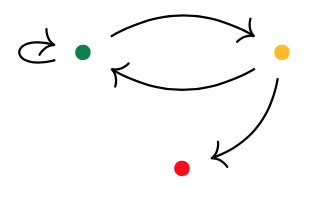Start at one vertex and move after a minute along a directed edge to the next (possibly the same) vertex. The potential evolutions in time will then form a tree, with each node given a label in $p(1)$.

If we start at the green dot, we get this tree of potential time-evolutions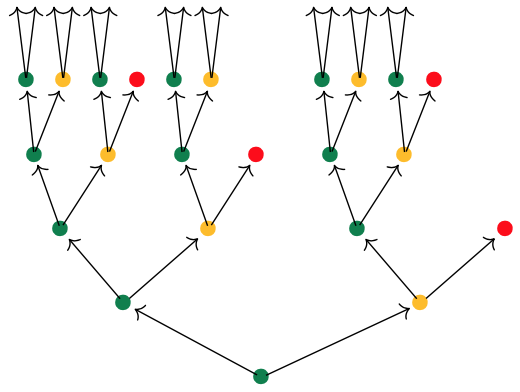There are exactly $\# p[i]$ branches leaving a node labeled $i \in p(1)$, and all subtrees emanating from equal labelled nodes are isomorphic.

If we had started at the yellow dot we had obtained a labelled tree isomorphic to the subtree emanating here from any yellow dot.

We can do the same things for any morphism in $\mathbf{Poly}$ of the form
$\varphi = (\varphi_1,\varphi[-])~:~Sy^S \rightarrow p$
Now, we have a directed graph with vertices the elements $s \in S$, with as many edges leaving vertex $s$ as there are elements $a \in p[\varphi_1(s)]$, and with the target vertex of the edge labeled $a$ starting in $s$ the vertex $\varphi[\varphi_1(s)](A)$.

Once we have this directed graph on $\# S$ vertices we can label vertex $s$ with the label $\varphi_1(s)$ from $p(1)$.

In this way, the time evolutions starting at a vertex $s \in S$ will give us a $p(1)$-labelled rooted tree.

But now, it is possibly that two distinct vertices can have the same $p(1)$-labeled tree of evolutions. But also, trees corresponding to equal labeled vertices can be different.

Right, I guess we’re ready to define the graph $G_{AB}$ and prove that $\mathbf{Learn(A,B)}$ is a topos.

In the case of learners, we have the target polynomial functor $p=C y^{A \times B}$ with $C = Maps(A,B) \times Maps(A \times B,A)$, that is
$p(1) = C \quad \text{and all} \quad p[i]=A \times B$

Start with the free rooted tree $T$ having exactly $\# A \times B$ branches growing from each node.

Here’s the directed graph $G_{AB}$:

• vertices $v_{\chi}$ correspond to the different $C$-labelings of $T$, one $C$-labeled rooted tree $T_{\chi}$ for every map $\chi : vtx(T) \rightarrow C$,
• arrows $v_{\chi} \rightarrow v_{\omega}$ if and only if $T_{\omega}$ is the rooted $C$-labelled tree isomorphic to the subtree of $T_{\chi}$ rooted at one step from the root.

A learner $\mathbf{A \rightarrow B}$ gives a set-valued representation of $\mathbf{G_{AB}}$.

We saw that a learner $A \rightarrow B$ is the same thing as a morphism in $\mathbf{Poly}$
$\varphi = (\varphi_1,\varphi[-])~:~P y^P \rightarrow C y^{A \times B}$
with $P$ the parameter set of maps.

Here’s what we have to do:

1. Draw the directed graph on vertices $p \in P$ giving the dynamics of the morphism $\varphi$. This graph describes how the learner can cycle through the parameter-set.

2. Use the map $\varphi_1$ to label the vertices with elements from $C$.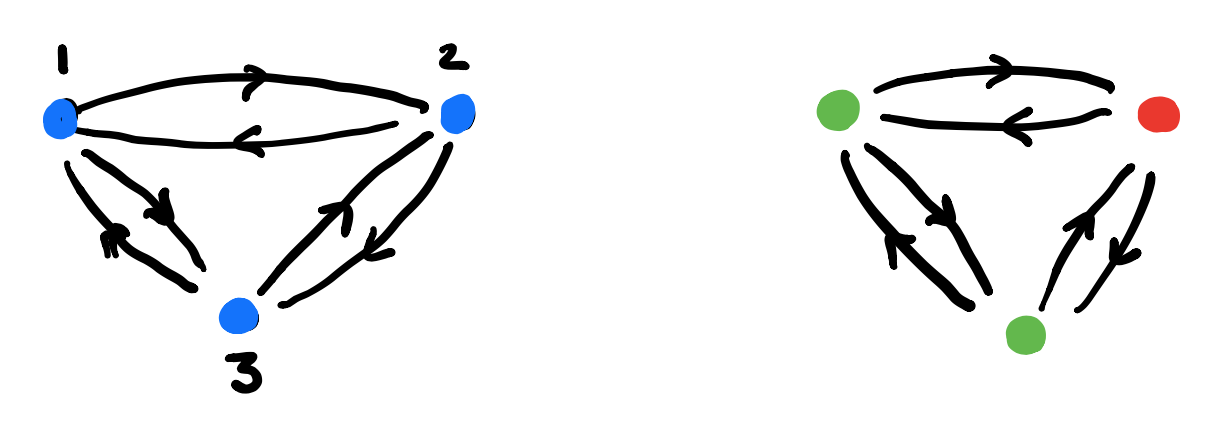3. For each vertex draw the rooted $C$-labeled tree of potential time-evolutions starting in that vertex.

In this example the time-evolutions of the two green vertices are the same, but in general they can be different.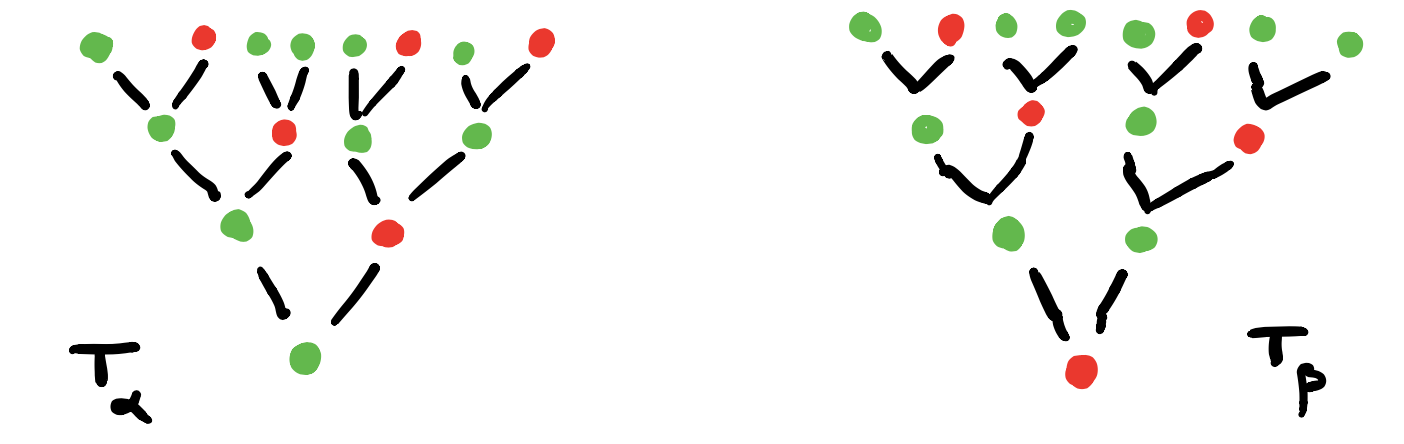4. Find the vertices in $G_{AB}$ determined by these $C$-labeled trees and note that they span a full subgraph of $G_{AB}$.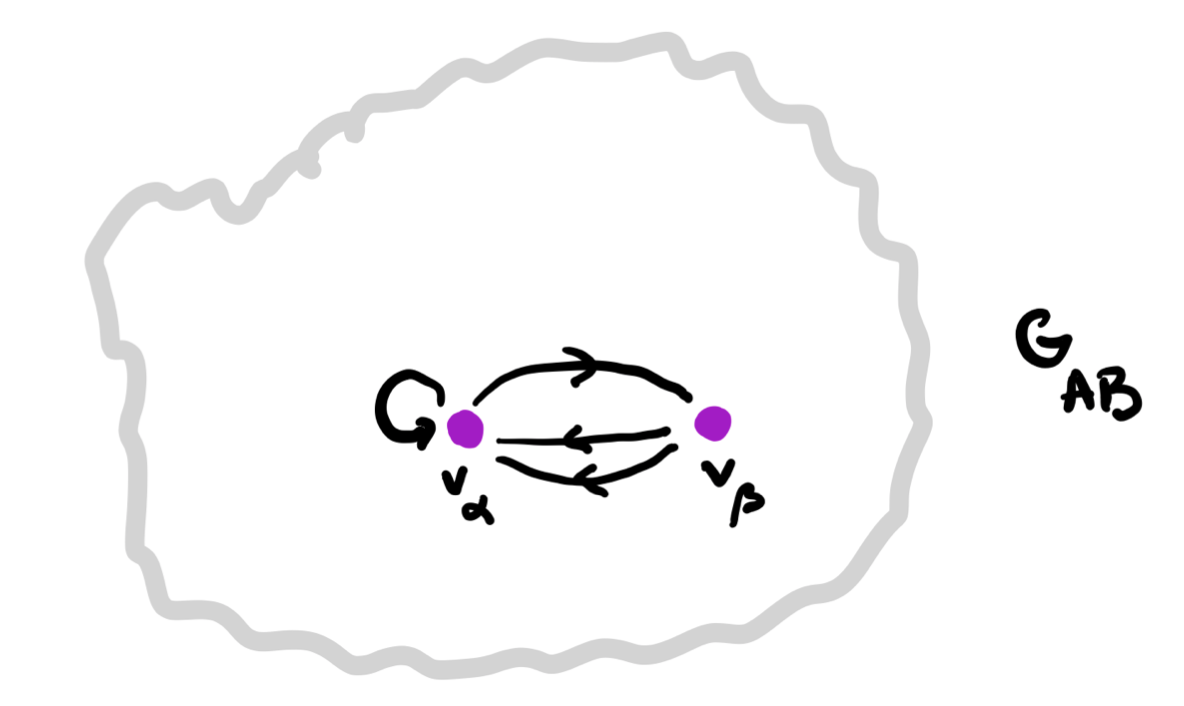5. The vertex-set $P_v$ consists of all elements from $p$ whose ($C$-labeled) vertex has evolution-tree $T_v$. If $v \rightarrow w$ is a directed edge in $G_{AB}$ corresponding to an element $(a,b) \in A \times B$, then the map on the vertex-sets corresponding to this edge is
$f_{v,(a,b)}~:~P_v \rightarrow P_w \qquad p \mapsto \varphi[\varphi_1(p)](a,b)$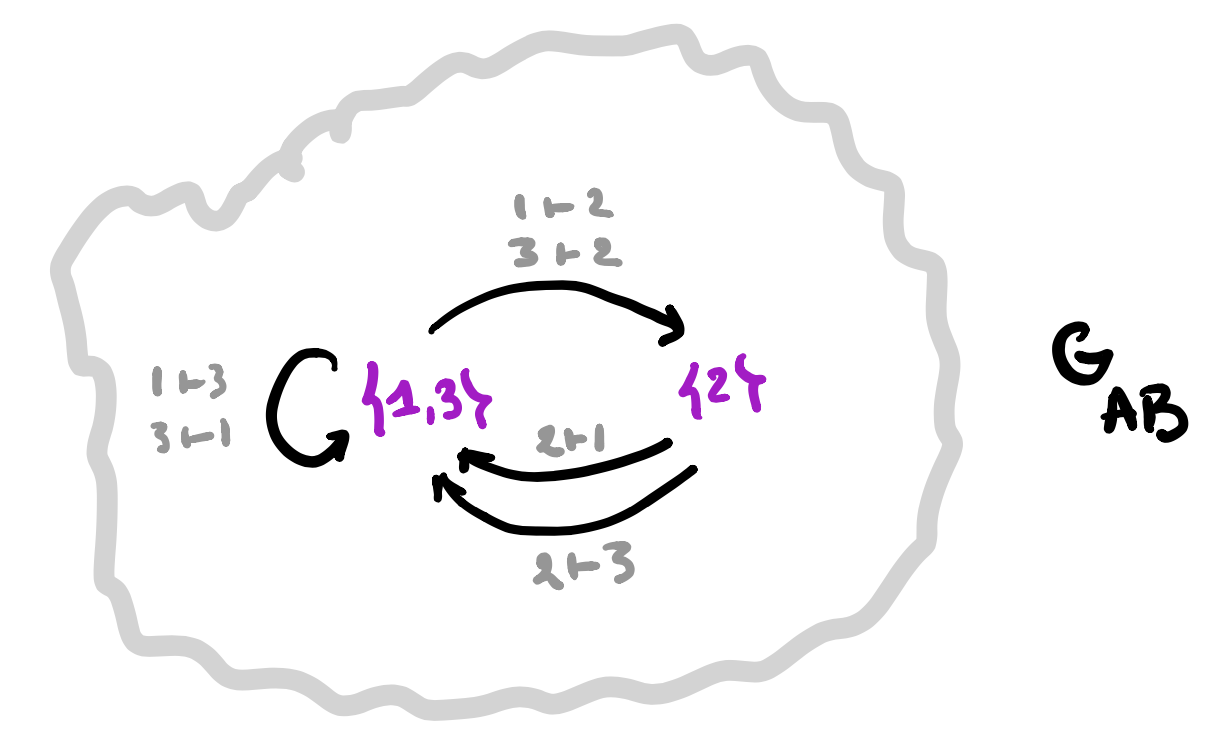A set-valued representation of $\mathbf{G_{AB}}$ gives a learner $\mathbf{A \rightarrow B}$.

1. Take a set-valued representation of $G_{AB}$, that is, the finite or infinite collection of vertices $V$ in $G_{AB}$ where the vertex-set $P_v$ is non-empty. Note that these vertices span a full subgraph of $G_{AB}$.

And, for each directed arrow $v \rightarrow w$ in this subgraph, labeled by an element $(a,b) \in A \times B$ we have a map
$f_{v,(a,b)}~:~P_v \rightarrow P_w$

2. The parameter set of our learner will be $P = \sqcup_v P_v$, the disjoint union of the non-empty vertex-sets.

3. The space-map $\varphi_1 : P \rightarrow C$ will send an element in $P_v$ to the $C$-label of the root of the tree $T_v$. This gives us already the interpretation and request maps
$I : P \times A \rightarrow B \quad \text{and} \quad R : P \times A \times B \rightarrow A$

4. The update map $U : P \times A \times B \rightarrow P$ follows from the sheaf-map we can define stalk-wise
$\varphi[\varphi_1(p)](a,b) = f_{v,(a,b)}(p)$
if $p \in P_v$.

That’s all folks!

$\mathbf{Learn(A,B)}$ is equivalent to the (covariant) functors $\mathbf{G_{AB} \rightarrow Sets}$.

Changing the directions of all arrows in $G_{AB}$ any covariant functor $\mathbf{G_{AB} \rightarrow Sets}$ becomes a contravariant functor $\mathbf{G_{AB}^o \rightarrow Sets}$, making $\mathbf{Learn(A,B)}$ an honest to Groth topos!

Every topos comes with its own logic, so we have a ‘learners’ logic’. (to be continued)

Following up on the deep learning and toposes-post, I was planning to do something on the logic of neural networks.

Prepping for this I saw David Spivak’s paper Learner’s Languages doing exactly that, but in the more general setting of ‘learners’ (see also the deep learning post).

And then … I fell under the spell of $\mathbf{Poly}$.

Spivak is a story-telling talent. A long time ago I copied his short story (actually his abstract for a talk) “Presheaf, the cobbler” in the Children have always loved colimits-post.

Last week, he did post Poly makes me happy and smart on the blog of the Topos Institute, which is another great read.

If this is way too ‘fluffy’ for you, perhaps you should watch his talk Poly: a category of remarkable abundance.

If you like (applied) category theory and have some days to waste, you can binge-watch all 15 episodes of the Poly-course Polynomial Functors: A General Theory of Interaction.

If you are more the reading-type, the 273 pages of the Poly-book will also kill a good number of your living hours.

Personally, I have no great appetite for category theory, I prefer to digest it in homeopathic doses. And, I’m allergic to co-terminology.

So then, how to define $\mathbf{Poly}$ for the likes of me?

$\mathbf{Poly}$, you might have surmised, is a category. So, we need ‘objects’ and ‘morphisms’ between them.

Any set $A$ has a corresponding ‘representable functor’ sending a given set $S$ to the set of all maps from $A$ to $S$
$y^A~:~\mathbf{Sets} \rightarrow \mathbf{Sets} \qquad S \mapsto S^A=Maps(A,S)$
This looks like a monomial in a variable $y$ ($y$ for Yoneda, of course), but does it work?

What is $y^1$, where $1$ stands for the one-element set $\{ \ast \}$? $Maps(1,S)=S$, so $y^1$ is the identity functor sending $S$ to $S$.

What is $y^0$, where $0$ is the empty set $\emptyset$? Well, for any set $S$ there is just one map $\emptyset \rightarrow S$, so $y^0$ is the constant functor sending any set $S$ to $1$. That is, $y^0=1$.

Going from monomials to polynomials we need an addition. We add such representable functors by taking disjoint unions (finite or infinite), that is
$\sum_{i \in I} y^{A_i}~:~\mathbf{Sets} \rightarrow \mathbf{Sets} \qquad S \mapsto \bigsqcup_{i \in I} Maps(A_i,S)$
If all $A_i$ are equal (meaning, they have the same cardinality) we use the shorthand $Iy^A$ for this sum.

The objects in $\mathbf{Poly}$ are exactly these ‘polynomial functors’
$p = \sum_{i \in I} y^{p[i]}$
with all $p[i] \in \mathbf{Sets}$. Remark that $p(1)=I$ as for any set $A$ there is just one map to $1$, that is $y^A(1) = Maps(A,1) = 1$, and we can write
$p = \sum_{i \in p(1)} y^{p[i]}$
An object $p \in \mathbf{Poly}$ is thus described by the couple $(p(1),p[-])$ with $p(1)$ a set, and a functor $p[-] : p(1) \rightarrow \mathbf{Sets}$ where $p(1)$ is now a category with objects the elements of $p(1)$ and no morphisms apart from the identities.

We can depict $p$ by a trimmed down forest, Spivak calls it the corolla of $p$, where the tree roots are the elements of $p(1)$ and the tree with root $i \in p(1)$ has one branch from the root for any element in $p[i]$. The corolla of $p=y^2+2y+1$ looks like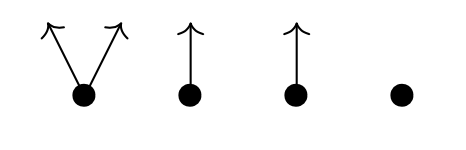If $M$ is an $m$-dimensional manifold, then you might view its tangent bundle $TM$ set-theoretically as the ‘corolla’ of the polynomial functor $M y^{\mathbb{R}^m}$, the tree-roots corresponding to the points of the manifold, and the branches to the different tangent vectors in these points.

Morphisms in $\mathbf{Poly}$ are a bit strange. For two polynomial functors $p=(p(1),p[-])$ and $q=(q(1),q[-])$ a map $p \rightarrow q$ in $\mathbf{Poly}$ consists of

• a map $\phi_1 : p(1) \rightarrow q(1)$ on the tree-roots in the right direction, and
• for any $i \in p(1)$ a map $q[\phi_1(i)] \rightarrow p[i]$ on the branches in the opposite direction

In our manifold/tangentbundle example, a morphism $My^{\mathbb{R}^m} \rightarrow y^1$ sends every point $p \in M$ to the unique root of $y^1$ and the unique branch in $y^1$ picks out a unique tangent-vector for every point of $M$. That is, vectorfields on $M$ are very special (smooth) morphisms $Mu^{\mathbb{R}^m} \rightarrow y^1$ in $\mathbf{Poly}$.

A smooth map between manifolds $M \rightarrow N$, does not determine a morphism $My^{\mathbb{R}^m} \rightarrow N y^{\mathbb{R}^n}$ in $\mathbf{Poly}$ because tangent vectors are pushed forward, not pulled back.

If instead we view the cotangent bundle $T^*M$ as the corolla of the polynomial functor $My^{\mathbb{R}^m}$, then everything works well.

But then, I promised not to use co-terminology…

Another time I hope to tell you how $\mathbf{Poly}$ helps us to understand the logic of learners.

Wednesday, Alexander Smirnov (Steklov Institute) gave the first talk in the $\mathbb{F}_1$ world seminar. Here’s his title and abstract:

Title: The 10th Discriminant and Tensor Powers of $\mathbb{Z}$

“We plan to discuss very shortly certain achievements and disappointments of the $\mathbb{F}_1$-approach. In addition, we will consider a possibility to apply noncommutative tensor powers of $\mathbb{Z}$ to the Riemann Hypothesis.”

Here’s his talk, and part of the comments section:

Smirnov urged us to pay attention to a 1933 result by Max Deuring in Imaginäre quadratische Zahlkörper mit der Klassenzahl 1:

“If there are infinitely many imaginary quadratic fields with class number one, then the RH follows.”

Of course, we now know that there are exactly nine such fields (whence there is no ‘tenth discriminant’ as in the title of the talk), and one can deduce anything from a false statement.

Deuring’s argument, of course, was different:

The zeta function $\zeta_{\mathbb{Q} \sqrt{-d}}(s)$ of a quadratic field $\mathbb{Q}\sqrt{-d}$, counts the number of ideals $\mathfrak{a}$ in the ring of integers of norm $n$, that is
$\sum_n \#(\mathfrak{a}:N(\mathfrak{a})=n) n^{-s}$
It is equal to $\zeta(s). L(s,\chi_d)$ where $\zeta(s)$ is the usual Riemann function and $L(s,\chi_d)$ the $L$-function of the character $\chi_d(n) = (\frac{-4d}{n})$.

Now, if the class number of $\mathbb{Q}\sqrt{-d}$ is one (that is, its ring of integers is a principal ideal domain) then Deuring was able to relate $\zeta_{\mathbb{Q} \sqrt{-d}}(s)$ to $\zeta(2s)$ with an error term, depending on $d$, and if we could run $d \rightarrow \infty$ the error term vanishes.

So, if there were infinitely many imaginary quadratic fields with class number one we would have the equality
$\zeta(s) . \underset{\rightarrow}{lim}~L(s,\chi_d) = \zeta(2s)$
Now, take a complex number $s \not=1$ with real part strictly greater that $\frac {1}{2}$, then $\zeta(2s) \not= 0$. But then, from the equality, it follows that $\zeta(s) \not= 0$, which is the RH.

To extend (a version of) the Deuring-argument to the $\mathbb{F}_1$-world, Smirnov wants to have many examples of commutative rings $A$ whose multiplicative monoid $A^{\times}$ is isomorphic to $\mathbb{Z}^{\times}$, the multiplicative monoid of the integers.

What properties must $A$ have?

Well, it can only have two units, it must be a unique factorisation domain, and have countably many irreducible elements. For example, $\mathbb{F}_3[x_1,\dots,x_n]$ will do!

(Note to self: contemplate the fact that all such rings share the same arithmetic site.)

Each such ring $A$ becomes a $\mathbb{Z}$-module by defining a new addition $+_{new}$ on it via
$a +_{new} b = \sigma^{-1}(\sigma(a) +_{\mathbb{Z}} \sigma(b))$
where $\sigma : A^{\times} \rightarrow \mathbb{Z}^{\times}$ is the isomorphism of multiplicative monoids, and on the right hand side we have the usual addition on $\mathbb{Z}$.

But then, any pair $(A,A’)$ of such rings will give us a module over the ring $\mathbb{Z} \boxtimes_{\mathbb{Z}^{\times}} \mathbb{Z}$.

It was not so clear to me what this ring is (if you know, please drop a comment), but I guess it must be a commutative ring having all these properties, and being a quotient of the ring $\mathbb{Z} \boxtimes_{\mathbb{F}_1} \mathbb{Z}$, the coordinate ring of the elusive arithmetic plane
$\mathbf{Spec}(\mathbb{Z}) \times_{\mathbf{Spec}(\mathbb{F}_1)} \mathbf{Spec}(\mathbb{Z})$

Smirnov’s hope is that someone can use a Deuring-type argument to prove:

“If $\mathbb{Z} \boxtimes_{\mathbb{Z}^{\times}} \mathbb{Z}$ is ‘sufficiently complicated’, then the RH follows.”

If you want to attend the seminar when it happens, please register for the seminar’s mailing list.

Judging from this and that paper, deep learning is the string theory of the 2020s for geometers and representation theorists.

If you want to know quickly what neural networks really are, I can recommend the post demystifying deep learning.

The typical layout of a deep neural network has an input layer $L_0$ allowing you to feed $N_0$ numbers to the system (a vector $\vec{v_0} \in \mathbb{R}^{N_0}$), an output layer $L_p$ spitting $N_p$ numbers back (a vector $\vec{v_p} \in \mathbb{R}^{N_p}$), and $p-1$ hidden layers $L_1,\dots,L_{p-1}$ where all the magic happens. The hidden layer $L_i$ has $N_i$ virtual neurons, their states giving a vector $\vec{v_i} \in \mathbb{R}^{N_i}$.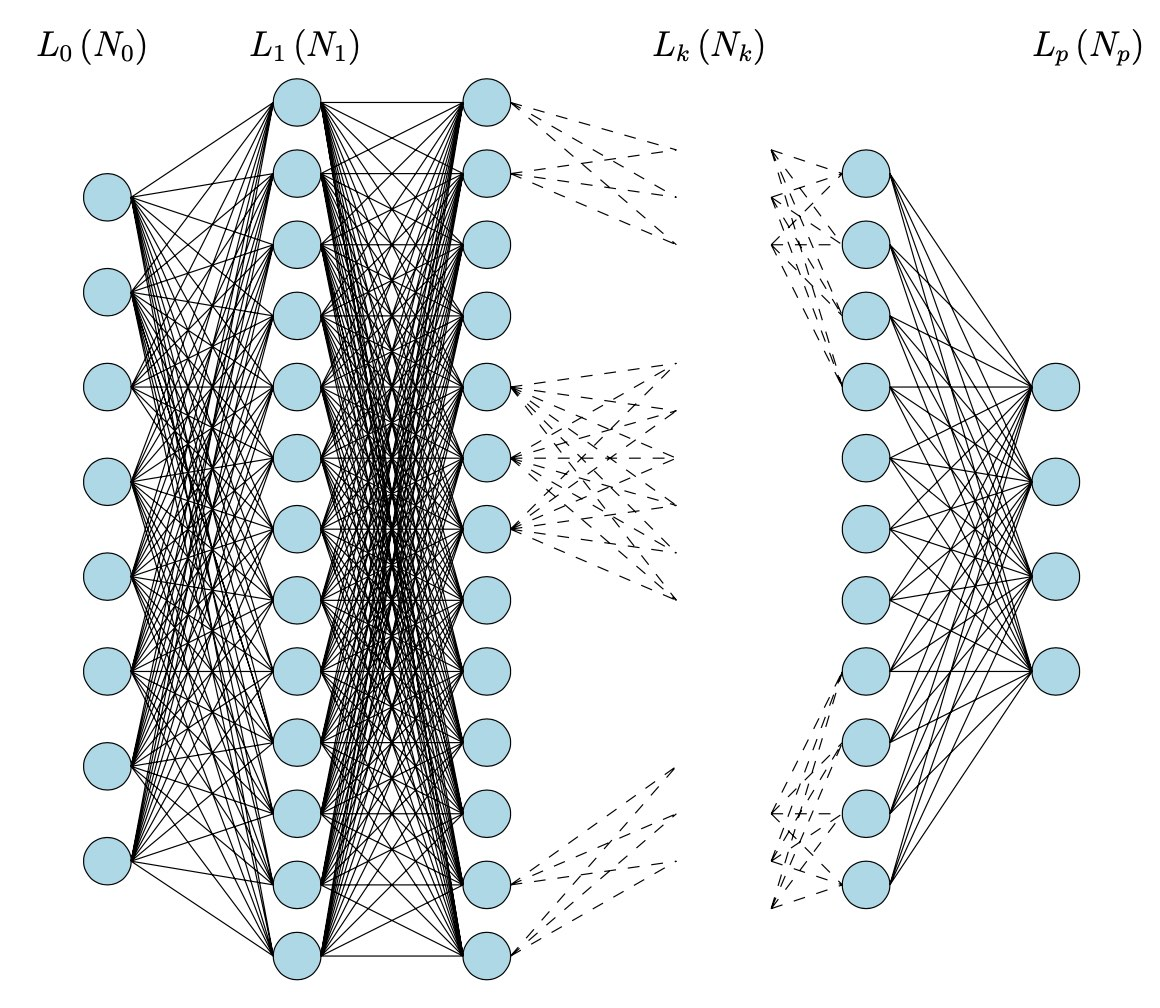Picture taken from Logical informations cells I

For simplicity let’s assume all neurons in layer $L_i$ are wired to every neuron in layer $L_{i+1}$, the relevance of these connections given by a matrix of weights $W_i \in M_{N_{i+1} \times N_i}(\mathbb{R})$.

If at any given moment the ‘state’ of the neural network is described by the state-vectors $\vec{v_1},\dots,\vec{v_{p-1}}$ and the weight-matrices $W_0,\dots,W_p$, then an input $\vec{v_0}$ will typically result in new states of the neurons in layer $L_1$ given by

$\vec{v_1}’ = c_0(W_0.\vec{v_0}+\vec{v_1})$

which will then give new states in layer $L_2$

$\vec{v_2}’ = c_1(W_1.\vec{v_1}’+\vec{v_2})$

and so on, rippling through the network, until we get as the output

$\vec{v_p} = c_{p-1}(W_{p-1}.\vec{v_{p-1}}’)$

where all the $c_i$ are fixed smooth activation functions $c_i : \mathbb{R}^{N_{i+1}} \rightarrow \mathbb{R}^{N_{i+1}}$.

This is just the dynamic, or forward working of the network.

The learning happens by comparing the computed output with the expected output, and working backwards through the network to alter slightly the state-vectors in all layers, and the weight-matrices between them. This process is called back-propagation, and involves the gradient descent procedure.

Even from this (over)simplified picture it seems doubtful that set valued (!) toposes are suitable to describe deep neural networks, as the Paris-Huawei-topos-team claims in their recent paper Topos and Stacks of Deep Neural Networks.

Still, there is a vast generalisation of neural networks: learners, developed by Brendan Fong, David Spivak and Remy Tuyeras in their paper Backprop as Functor: A compositional perspective on supervised learning (which btw is an excellent introduction for mathematicians to neural networks).

For any two sets $A$ and $B$, a learner $A \rightarrow B$ is a tuple $(P,I,U,R)$ where

• $P$ is a set, a parameter space of some functions from $A$ to $B$.
• $I$ is the interpretation map $I : P \times A \rightarrow B$ describing the functions in $P$.
• $U$ is the update map $U : P \times A \times B \rightarrow P$, part of the learning procedure. The idea is that $U(p,a,b)$ is a map which sends $a$ closer to $b$ than the map $p$ did.
• $R$ is the request map $R : P \times A \times B \rightarrow A$, the other part of the learning procedure. The idea is that the new element $R(p,a,b)=a’$ in $A$ is such that $p(a’)$ will be closer to $b$ than $p(a)$ was.

The request map is also crucial is defining the composition of two learners $A \rightarrow B$ and $B \rightarrow C$. $\mathbf{Learn}$ is the (symmetric, monoidal) category with objects all sets and morphisms equivalence classes of learners (defined in the natural way).

In this way we can view a deep neural network with $p$ layers as before to be the composition of $p$ learners
$\mathbb{R}^{N_0} \rightarrow \mathbb{R}^{N_1} \rightarrow \mathbb{R}^{N_2} \rightarrow \dots \rightarrow \mathbb{R}^{N_p}$
where the learner describing the transition from the $i$-th to the $i+1$-th layer is given by the equivalence class of data $(A_i,B_i,P_i,I_i,U_i,R_i)$ with
$A_i = \mathbb{R}^{N_i},~B_i = \mathbb{R}^{N_{i+1}},~P_i = M_{N_{i+1} \times N_i}(\mathbb{R}) \times \mathbb{R}^{N_{i+1}}$
and interpretation map for $p = (W_i,\vec{v}_{i+1}) \in P_i$
$I_i(p,\vec{v_i}) = c_i(W_i.\vec{v_i}+\vec{v}_{i+1})$
The update and request maps (encoding back-propagation and gradient-descent in this case) are explicitly given in theorem III.2 of the paper, and they behave functorial (whence the title of the paper).

More generally, we will now associate objects of a topos (actually just sheaves over a simple topological space) to a network op $p$ learners
$A_0 \rightarrow A_1 \rightarrow A_2 \rightarrow \dots \rightarrow A_p$
inspired by section I.2 of Topos and Stacks of Deep Neural Networks.

The underlying category will be the poset-category (the opposite of the ordering of the layers)
$0 \leftarrow 1 \leftarrow 2 \leftarrow \dots \leftarrow p$
The presheaf on a poset is a locale and in this case even the topos of sheaves on the topological space with $p+1$ nested open sets.
$X = U_0 \supseteq U_1 \supseteq U_2 \supseteq \dots \supseteq U_p = \emptyset$
If the learner $A_i \rightarrow A_{i+1}$ is (the equivalence class) of the tuple $(A_i,A_{i+1},P_i,I_i,U_i,R_i)$ we will now describe two sheaves $\mathcal{W}$ and $\mathcal{X}$ on the topological space $X$.

$\mathcal{W}$ has as sections $\Gamma(\mathcal{W},U_i) = \prod_{j=i}^{p-1} P_i$ and the obvious projection maps as the restriction maps.

$\mathcal{X}$ has as sections $\Gamma(\mathcal{X},U_i) = A_i \times \Gamma(\mathcal{W},U_i)$ and restriction map to the next smaller open
$\rho^i_{i+1}~:~\Gamma(\mathcal{X},U_i) \rightarrow \Gamma(\mathcal{X},U_{i+1}) \qquad (a_i,(p_i,p’)) \mapsto (p_i(a_i),p’)$
and other retriction maps by composition.

A major result in Topos and Stacks of Deep Neural Networks is that back-propagation is a natural transformation, that is, a sheaf-morphism $\mathcal{X} \rightarrow \mathcal{X}$.

In this general setting of layered learners we can always define a map on the sections of $\mathcal{X}$ (for every open $U_i$), $\Gamma(\mathcal{X},U_i) \rightarrow \Gamma(\mathcal{X},U_i)$
$(a_,(p_i,p’)) \mapsto (R(p_i,a_i,p_i(a_i)),(U(p_i,a_i,p_i(a_i)),p’)$
But, in order for this to define a sheaf-morphism, compatible with the restrictions, we will have to impose restrictions on the update and restriction maps of the learners, in general.

Still, in the special case of deep neural networks, this compatibility follows from the functoriality property of Backprop as Functor: A compositional perspective on supervised learning.

To be continued.

In 2017-18, the seminar Lectures grothendieckiennes took place at the ENS in Paris. Among the speakers were Alain Connes, Pierre Cartier, Laurent Lafforgue and Georges Maltsiniotis.

Olivia Caramello, who also contributed to the seminar, posts on her blog Around Toposes that the proceedings of this lectures series is now available from the SMF.

Olivia’s blogpost links also to the YouTube channel of the seminar. Several of these talks are well worth your time watching.

If you are at all interested in toposes and their history, and if you have 90 minutes to kill, I strongly recommend watching Colin McLarthy’s talk Grothendieck’s 1973 topos lectures:

In 1973, Grothendieck gave three lectures series at the Department of Mathematics of SUNY at Buffalo, the first on ‘Algebraic Geometry’, the second on ‘The Theory of Algebraic Groups’ and the third one on ‘Topos Theory’.

All of these Grothendieck talks were audio(!)-taped by John (Jack) Duskin, who kept and preserved them with the help of William Lawvere. They constitute more than 100 hours of rare recordings of Grothendieck.

“The copyright of all these recordings is that of the Department of Mathematics of SUNY at Buffalo to whose representatives, in particular Professors Emeritus Jack DUSKIN and Bill LAWVERE exceptional thanks are due both for the preservation and transmission of this historic archive, the only substantial archive of recordings of courses given by one of the greatest mathematicians of all time, whose work and ideas exercised arguably the most profound influence of any individual figure in shaping the mathematics of the second half od the 20th Century. The material which it is proposed to make available here, with their agreement, will form a mirror site to the principal site entitled “Grothendieck at Buffalo” (url: ).”

Sadly, the URL is still missing.

Fortunately, another answer links to the Grothendieck project Thèmes pour une Harmonie by Mateo Carmona. If you scroll down to the 1973-section, you’ll find there all of the recordings of these three Grothendieck series of talks!

To whet your appetite, here’s the first part of his talk on topos theory on April 4th, 1973:

For all subsequent recordings of his talks in the Topos Theory series on May 11th, May 18th, May 25th, May 30th, June 4th, June 6th, June 20th, June 27th, July 2nd, July 10th, July 11th and July 12th, please consult Mateo’s website (under section 1973).

For some time I knew it was in the making, now they are ready to launch it:

The $\mathbb{F}_1$ World Seminar, an online seminar dedicated to the “field with one element”, and its many connections to areas in mathematics such as arithmetic, geometry, representation theory and combinatorics. The organisers are Jaiung Jun, Oliver Lorscheid, Yuri Manin, Matt Szczesny, Koen Thas and Matt Young.

From the announcement:

“While the origins of the “$\mathbb{F}_1$-story” go back to attempts to transfer Weil’s proof of the Riemann Hypothesis from the function field case to that of number fields on one hand, and Tits’s Dream of realizing Weyl groups as the $\mathbb{F}_1$ points of algebraic groups on the other, the “$\mathbb{F}_1$” moniker has come to encompass a wide variety of phenomena and analogies spanning algebraic geometry, algebraic topology, arithmetic, combinatorics, representation theory, non-commutative geometry etc. It is therefore impossible to compile an exhaustive list of topics that might be discussed. The following is but a small sample of topics that may be covered:

Algebraic geometry in non-additive contexts – monoid schemes, lambda-schemes, blue schemes, semiring and hyperfield schemes, etc.
Arithmetic – connections with motives, non-archimedean and analytic geometry
Tropical geometry and geometric matroid theory
Algebraic topology – K-theory of monoid and other “non-additive” schemes/categories, higher Segal spaces
Representation theory – Hall algebras, degenerations of quantum groups, quivers
Combinatorics – finite field and incidence geometry, and various generalizations”

The seminar takes place on alternating Wednesdays from 15:00 PM – 16:00 PM European Standard Time (=GMT+1). There will be room for mathematical discussion after each lecture.

The first meeting takes place Wednesday, January 19th 2022. If you want to receive abstracts of the talks and their Zoom-links, you should sign up for the mailing list.

Perhaps I’ll start posting about $\mathbb{F}_1$ again, either here, or on the dormant $\mathbb{F}_1$ mathematics blog. (see this post for its history).